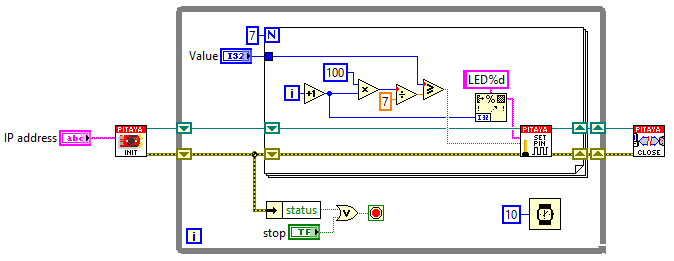# 2.3.1.4.1.4. Interactive LED bar graph¶

## 2.3.1.4.1.4.1. Description¶

This example shows how to make a bar graph by controlling Red Pitaya on board LEDs. The number of LEDs that will be turned ON, corresponds to the value of variable p that can be set by MATLAB® slider bar.

## 2.3.1.4.1.4.2. Required hardware¶

• Red Pitaya device## 2.3.1.4.1.4.3. Code - MATLAB®¶

The code is written in MATLAB. In the code we use SCPI commands and TCP/IP communication. Copy code from below to MATLAB editor, input value p save project and press run. Change p with slider bar from 0-100.

```function sliderDemo

f = figure(1);
global p

%// initialize the slider
h = uicontrol(...
'parent'  , f,...
'units'   , 'normalized',...                 %// pixels settings
'style'   , 'slider',...
'position', [0.05 0.05 0.9 0.05],...
'min'     , 1,...                            %// Make the "value" between min ...
'max'     , 100,...                          %// max 10, with initial value
'value'   , 10,...                           %// as set.
'callback', @sliderCallback);                %// This is called when using the
%// arrows
%// and/or when clicking the slider bar

hLstn = handle.listener(h,'ActionEvent',@sliderCallback);
%// (variable appears unused, but not assigning it to anything means that
%// the listener is stored in the 'ans' variable. If "ans" is overwritten,
%// the listener goes out of scope and is thus destroyed, and thus, it no
%// longer works.

function  sliderCallback(~,~)

p =(get(h,'value'))

% Define Red Pitaya as TCP/IP object

IP= '192.168.178.56';           % Input IP of your Red Pitaya...
port = 5000;
tcpipObj=tcpip(IP, port);

%% Open connection with your Red Pitaya

fopen(tcpipObj);
tcpipObj.Terminator = 'CR/LF';

if p >=(100/7)
fprintf(tcpipObj,'DIG:PIN LED1,1')
else
fprintf(tcpipObj,'DIG:PIN LED1,0')
end

if p >=(100/7)*2
fprintf(tcpipObj,'DIG:PIN LED2,1')
else
fprintf(tcpipObj,'DIG:PIN LED2,0')
end

if p >=(100/7)*3
fprintf(tcpipObj,'DIG:PIN LED3,1')
else
fprintf(tcpipObj,'DIG:PIN LED3,0')
end

if p >=(100/7)*4
fprintf(tcpipObj,'DIG:PIN LED4,1')
else
fprintf(tcpipObj,'DIG:PIN LED4,0')
end

if p >=(100/7)*5
fprintf(tcpipObj,'DIG:PIN LED5,1')
else
fprintf(tcpipObj,'DIG:PIN LED5,0')
end

if p >=(100/7)*6
fprintf(tcpipObj,'DIG:PIN LED6,1')
else
fprintf(tcpipObj,'DIG:PIN LED6,0')
end

if p >=(100/7)*7
fprintf(tcpipObj,'DIG:PIN LED7,1')
else
fprintf(tcpipObj,'DIG:PIN LED7,0')
end

if p >=(100/8)*7
fprintf(tcpipObj,'DIG:PIN LED8,1')
else
fprintf(tcpipObj,'DIG:PIN LED8,0')
end

fclose(tcpipObj);
end
end
```

## 2.3.1.4.1.4.4. Code - LabVIEW¶